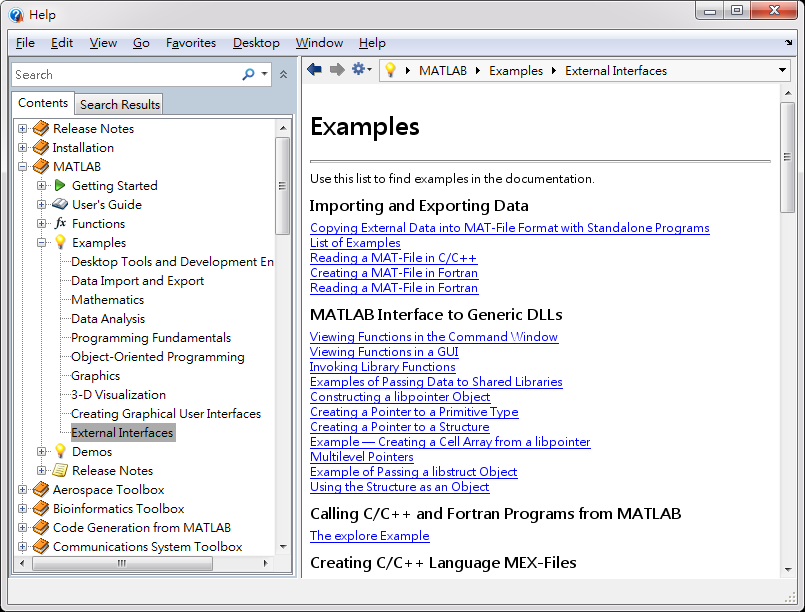## 3-1 MATLAB ?厩鍂蝔见?隞钅𢒰

MATLAB 提供了功能完整的應用程式介面（Application Program Interface，簡稱 API），來和外部程式或其它程式語言溝通，只要遵循 MATLAB 的 API 的規範，可以做到下列事項：

1. 從MATLAB 呼叫以 C 語言或 Fortran 語言撰寫的函數。
2. 將 MATLAB 當成一個計算引擎（Computing Engine），並從獨立的 C 或 Fortran 程式來呼叫此 MATLAB 引擎，進行各種運算或圖形顯示。
3. 以獨立（Standalone）的 C 或 Fortran 程式讀寫 MATLAB 專用的 MAT 檔案。
4. 在微軟的視窗環境下，進行 ActiveX Automation，亦即可將 MATLAB 視為 ActiveX Automation Server，來讓其它應用程式（例如：Microsoft Excel 或 Access）呼叫，或將 MATLAB 視為 ActiveX Automation Controller，來呼叫微軟視窗下其它的應用程式。
5. 在微軟的視窗環境下，經由 DDE（Dynamic Data Exchange）來和其它應用程式溝通並傳送資料。MATLAB程式設計：進階篇